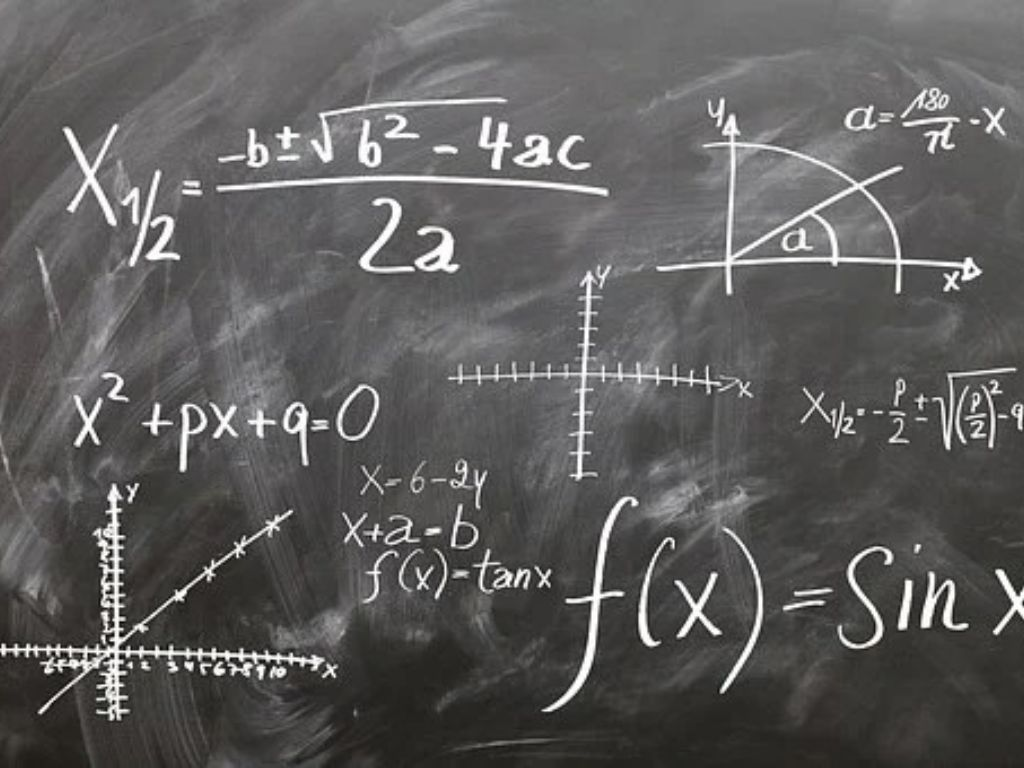# Quadratic Formula (Shridharacharya Sutra) Class 10th

## Introduction

Quadratic Formula (Shridharacharya Sutra) – The quadratic formula is given by the great Indian mathematician Shridharacharya and is also known as Shridharacharya Sutra. This formula is used to solve the quadratic equation.

x = –b ± √(b2 – 4ac)/2a

Where, x = variable

a, b, c = Coefficients of quadratic equation

### Derivation of Formula

This formula can be derived from the perfect square method.

The standard form of a quadratic equation is

ax2 + bx + c = 0

x2 + bx/a + c/a = 0  (taking 1 as coefficient of x2 or dividing the whole equation by the coefficient of x2 (a))

x2 + bx/a = −c/a        (taking the constant term to the right side)

x2 + bx/a + (b/2a)2 = −c/a + (b/2a)2   (adding square of half of coefficient of x)

(x + b/2a)2 = −c/a + b2/4a2

(x + b/2a)2 = (−c⨯4a + b2⨯1)/4a2 = (−4ac + b2)/4a2

Taking square root on both sides,

√(x + b/2a)2 = √(−4ac + b2)/4a2

x + b/2a = ±√(b2 − 4ac)/2a

x = −b/2a ± √(b2 − 4ac)/2a

x = −b ± √(b2 − 4ac)/2a

Here sign ± shows the two values of x.

x = −b + √(b2 − 4ac)/2a                           x = −b − √(b2 − 4ac)/2a

The above values of x are the Quadratic Formula (Shridharacharya sutra).

Example – Solve the quadratic equation 9x2 + 7x – 2 = 0 by Shridharacharya sutra.

Solution – given equation 9x2 + 7x – 2 = 0

Comparing with standard form ax2 + bx + c = 0

So, a = 9, b = 7, c = – 2

By quadratic formula,  x = −b ± √(b2 − 4ac)/2a

Putting the values of a, b, c

x = −7 ± √{72 − 4⨯9⨯(-2)}/2⨯9

x = −7 ± √{49 + 72}/18

x = −7 ± √{121}/18 = −7 ± 11/18

On taking the (+) sign,

x = −7 + 11/18

x = 4/18

x = 2/9

On taking the (−) sign,

x = −7 − 11/18

x = −18/18

x = −1

These values of x are the roots of the given quadratic equation.    Ans.

### Discriminant and Nature of Roots

We can find the nature of roots by the term b2 – 4ac used in the Shridharacharya sutra which is called the Discriminant. It is denoted by D.

D = b2 – 4ac

⇒ If D > 0 then the roots will be different and real.

x = (−b + √D)/2a       and       x = (−b − √D)/2a

⇒ If D = 0 then the roots will be equal and real.

x = −b/2a       and       x = −b/2a

⇒ If D < 0 then the roots will not be real, they will be imaginary.

#### Some Examples –

Example – 1) find the nature of the roots of equation 2x2 – 6x + 3 = 0 and if roots exist then find them.

Solution – Given equation 2x2 – 6x + 3 = 0

Comparing the equation with ax2 + bx + c = 0

Here, a = 2, b = – 6, c = 3

Now D = b2 – 4ac

D = (-6)2 – 4⨯2⨯3 = 36 – 24 = 12

D > 0

∴ The roots of equation 2x2 – 6x + 3 = 0 will be different and Real.

By Shridharacharya formula,   x = (−b ± √D)/2a        where, D = b2 – 4ac

x = {−(-6) ± √12)}/2⨯2 = {6 ± √(4⨯3)}/4

x = {6 ± 2√3}/4 = 2{3 ± √3}/4 = {3 ± √3}/2

So, the roots are x = (3 + √3)/2 and x = (3 − √3)/2.       Ans.

Example – 2) find the nature of roots of the equation x2 – 4x + 4 = 0 and if roots exist then find them.

Solution – Given equation x2 – 4x + 4 = 0

By comparing with ax2 + bx + c = 0

a = 1, b = – 4, c = 4

Now, D = b2 – 4ac

D = (-4)2 – 4⨯1⨯4 = 16 – 16 = 0

D = 0

∴ The roots of the equation x2 – 4x + 4 = 0 will be equal and Real.

By quadratic formula,   x = (−b ± √D)/2a        where, D = b2 – 4ac

x = {−(-4) ± √0)/2⨯1 = (4 ± 0)/2 = 4/2

x = 2

So, the roots are x = 2 and x = 2.       Ans.

Example – 3) find the nature of the roots of equation 2x2 + 3x + 5 = 0 and if roots exist then find them.

Solution – Given equation 2x2 + 3x + 5 = 0

On comparing with ax2 + bx + c = 0

a = 2, b = 3, c = 5

Now D = b2 – 4ac

D = (3)2 – 4⨯2⨯5 = 9 – 40 = –31

D < 0

∴ The roots of equation 2x2 + 3x + 5 = 0 will not be Real. They will be imaginary.

By Shridharacharya quadratic formula,   x = (−b ± √D)/2a       where, D = b2 – 4ac

x = (−3 ± √-31)/2⨯2 = (−3 ± √−1⨯31)/4

x = (−3 ± √−1⨯√31)/4 = (−3 ± √31i )/4

So, the roots x = (−3 + √31i )/4 and x = (−3 − √31i )/4.  where, i(iota) = √−1

Quadratic Formula (Shridharacharya Sutra) Class 10th in Hindi

Rate this post

### 6 thoughts on “Quadratic Formula (Shridharacharya Sutra) Class 10th”

1.Perfect blog, This blog is good for class 10 math students. It’s also have quadratic formulas.

2.Thank you for great information

3.Ηеllo every one, heгe everү person is sharing these know-how, thus it’s
fastidious to reаd thіs webpage, and I used to go to see thіѕ
website daily.